# elektronn3.data.transforms.transforms module¶

Transformations (data augmentation, normalization etc.) for semantic segmantation.

Important note: The transformations here have a similar interface to torchvision.transforms, but there are two key differences:

1. They all map (inp, target) pairs to (transformed_inp, transformed_target) pairs instead of just inp to transformed_inp. Most transforms don’t change the target, though.

2. They exclusively operate on numpy.ndarray data instead of PIL or torch.Tensor data.

class elektronn3.data.transforms.transforms.AdditiveGaussianNoise(sigma=0.1, channels=None, prob=1.0)[source]

Bases: object

Adds random gaussian noise to the input.

Parameters
• sigma (float) – Sigma parameter of the gaussian distribution to draw from

• channels (Optional[Sequence[int]]) – If channels is None, the noise is applied to all channels of the input tensor. If channels is a Sequence[int], noise is only applied to the specified channels.

• prob (float) – probability (between 0 and 1) with which to perform this augmentation. The input is returned unmodified with a probability of 1 - prob.

class elektronn3.data.transforms.transforms.AlbuSeg2d(albu)[source]

Bases: object

Wrapper for albumentations’ segmentation-compatible 2d augmentations.

Wraps an augmentation so it can be used within elektronn3’s transform pipeline. See https://github.com/albu/albumentations.

If target is None, it is ignored. Else, it is passed to the wrapped albumentations augmentation as the mask argument.

Parameters

albu (albumentations.core.transforms_interface.DualTransform) – albumentation object of type DualTransform.

Example:

>>> import albumentations
>>> transform = AlbuSeg2d(albumentations.ShiftScaleRotate(
...     p=0.98, rotate_limit=180, scale_limit=0.1, interpolation=2
... ))
... # Note that interpolation=2 means cubic interpolation (-> cv2.CUBIC constant).
... # Don't confuse this with scipy's interpolation options.

class elektronn3.data.transforms.transforms.Clahe2d[source]

Bases: object

class elektronn3.data.transforms.transforms.Compose(transforms)[source]

Bases: object

Composes several transforms together.

Parameters

transforms (list of Transform objects) – list of transforms to compose.

Example

>>> Compose([
>>>     Normalize(mean=(155.291411,), std=(41.812504,)),
>>> ])

class elektronn3.data.transforms.transforms.DistanceTransformTarget(scale=50.0, normalize_fn=<ufunc 'tanh'>, inverted=True, signed=True, vector=False)[source]

Bases: object

Converts discrete binary label target tensors to their (signed) euclidean distance transform (EDT) representation.

Based on the method proposed in https://arxiv.org/abs/1805.02718.

Parameters
• scale (Optional[float]) – Scalar value to divide distances before applying normalization

• normalize_fn (Optional[Callable[[ndarray], ndarray]]) – Function to apply to distance map for normalization.

• inverted (bool) – Invert target labels before computing transform if True. This means the distance map will show the distance to the nearest foreground pixel at each background pixel location (which is the opposite behavior of standard distance transform).

• signed (bool) – Compute signed distance transform (SEDT), where foreground regions are not 0 but the negative distance to the nearest foreground border.

• vector (bool) – Return distance vector map instead of scalars.

edt(target)[source]
Return type

ndarray

class elektronn3.data.transforms.transforms.DropIfTooMuchBG(bg_id=0, threshold=0.9, prob=1.0)[source]

Bases: object

Filter transform that skips a sample if the background class is too dominant in the target.

class elektronn3.data.transforms.transforms.ElasticTransform(sigma=4, alpha=40, channels=None, prob=0.25, target_discrete_ix=None, aniso_factor=1.0, draw_debug_grid=False)[source]

Bases: object

Elastic deformation of images as described in Simard, 2003 (DOI 10.1109/ICDAR.2003.1227801)

Parameters
• sigma (float) – Sigma parameter of the gaussian smoothing performed on the random displacement field. High sigma values (> 4) lead to less randomness and more spatial consistency.

• alpha (float) – Factor by which all random displacements are multiplied. Each local displacement is drawn from the range [-alpha, alpha], so e.g. for alpha=1 you won’t see much of an effect.

• channels (Optional[Sequence[int]]) – If channels is None, the change is applied to all channels of the input tensor. If channels is a Sequence[int], change is only applied to the specified channels.

• prob (float) – probability (between 0 and 1) with which to perform this augmentation. The input is returned unmodified with a probability of 1 - prob

• target_discrete_ix (Optional[Sequence[int]]) –

list List of target channels that contain discrete values. By default (None), every channel is is seen as discrete (this is generally the case for classification tasks). This information is used to decide what kind of interpolation should be used for reading target data:

• discrete targets are obtained by nearest-neighbor interpolation

• non-discrete (continuous) targets are linearly interpolated.

• aniso_factor (float) – Factor by which to divide the deformation strength in the z axis. E.g. if the data has half resolution in the z dimension, set aniso_factor = 2. By default it is 1, so every spatial dimension is treated equally.

• draw_debug_grid (bool) – If True, draw a 16-spaced grid into the image to visualize deformations. This is only for debugging purposes and should never be enabled during training.

The input image should be of dimensions (C, H, W) or (C, D, H, W). C must be included.

class elektronn3.data.transforms.transforms.Identity[source]

Bases: object

class elektronn3.data.transforms.transforms.Lambda(func)[source]

Bases: object

Wraps a function of the form f(x, y) = (x’, y’) into a transform.

Parameters

func (Callable[[ndarray, ndarray], Tuple[ndarray, ndarray]]) – A function that takes two arrays and returns a tuple of two arrays.

Example

>>> # Multiplies inputs (x) by 255, leaves target (y) unchanged
>>> t = Lambda(lambda x, y: (x * 255, y))

>>> # You can also pass regular Python functions to Lambda
>>> def flatten(x, y):
>>>     return x.reshape(-1), y.reshape(-1)
>>> t = Lambda(flatten)

class elektronn3.data.transforms.transforms.Normalize(mean, std, inplace=False)[source]

Bases: object

Normalizes inputs with supplied per-channel means and stds.

Parameters
• mean (Union[Sequence[float], float]) – Global mean value(s) of the inputs. Can either be a sequence of float values where each value corresponds to a channel or a single float value (only for single-channel data).

• std (Union[Sequence[float], float]) – Global standard deviation value(s) of the inputs. Can either be a sequence of float values where each value corresponds to a channel or a single float value (only for single-channel data).

• inplace (bool) – Apply in-place (works faster, needs less memory but overwrites inputs).

class elektronn3.data.transforms.transforms.RandomBlurring(config, patch_shape=None)[source]

Bases: object

class elektronn3.data.transforms.transforms.RandomBrightnessContrast(brightness_std=0.5, contrast_std=0.5, channels=None, prob=1.0)[source]

Bases: object

Randomly changes brightness and contrast of the input image.

Parameters
• brightness_std (float) – Standard deviation of contrast change strength (bias)

• contrast_std (float) – Standard deviation of brightness change strength (scale)

• channels (Optional[Sequence[int]]) – If channels is None, the change is applied to all channels of the input tensor. If channels is a Sequence[int], change is only applied to the specified channels.

• prob (float) – probability (between 0 and 1) with which to perform this augmentation. The input is returned unmodified with a probability of 1 - prob.

class elektronn3.data.transforms.transforms.RandomCrop(crop_shape)[source]

Bases: object

class elektronn3.data.transforms.transforms.RandomFlip(ndim_spatial=2)[source]

Bases: object

Randomly flips spatial input and target dimensions respectively. Spatial dimensions are considered to occur last in the input/target shape and are flipped with probability p=0.5 (iid).

Parameters

ndim_spatial (int) – Number of spatial dimension in input, e.g. ndim_spatial=2 for input shape (N, C, H, W)

class elektronn3.data.transforms.transforms.RandomGammaCorrection(gamma_std=0.5, gamma_min=0.25, channels=None, prob=1.0)[source]

Bases: object

Applies random gamma correction to the input.

Parameters
• gamma_std (float) – standard deviation of the gamma value.

• channels (Optional[Sequence[int]]) – If channels is None, the change is applied to all channels of the input tensor. If channels is a Sequence[int], change is only applied to the specified channels.

• prob (float) – probability (between 0 and 1) with which to perform this augmentation. The input is returned unmodified with a probability of 1 - prob.

class elektronn3.data.transforms.transforms.RandomGaussianBlur(distsigma=1, channels=None, prob=1.0, aniso_factor=None)[source]

Bases: object

Adds random gaussian blur to the input.

Parameters
• distsigma – Sigma parameter of the half-normal distribution from which sigmas for the gaussian blurring are drawn. To clear up possible confusion: The distsigma parameter does not directly parametrize the gaussian blurring, but the random distribution from which the blurring sigmas are drawn from.

• prob – probability (between 0 and 1) with which to perform this augmentation. The input is returned unmodified with a probability of 1 - prob.

• aniso_factor – a tuple or an array to apply the anisotropy, must match the dimension of the input.

class elektronn3.data.transforms.transforms.RandomGrayAugment(channels=None, prob=1.0)[source]

Bases: object

Performs gray value augmentations in the same way as ELEKTRONN2’s greyAugment() function, but with additional support for inputs outside of the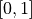intensity value range. Targets are not modified.

This augmentation method randomly selects the values alpha, beta and gamma within sensible ranges and subsequently performs:

• Temporarily rescaling image intensities to therange (necessary for gamma correction).

• Linear intensity scaling (contrast) by multiplying the input with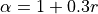, where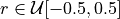. Value range: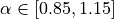.

• Adding a constant value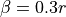, where. Value range: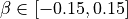.

• Gamma correction with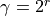, where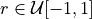.

• Clipping all image intensity values to the range.

• Re-rescaling intensities back to the original input value range.

class elektronn3.data.transforms.transforms.RandomRotate2d(angle_range=- 180, 180, prob=1)[source]

Bases: object

Random rotations in the xy plane, based on scikit-image.

If inputs are 3D images ([C,] D, H, W) Rotate them as a stack of 2D images by the same angle, constraining the rotation direction to the xy plane

class elektronn3.data.transforms.transforms.RandomSlicewiseTransform(transform, prob=0.1, inplace=True)[source]

Bases: object

Wraps any 2D transform and applies it per 2D slice independently in a 3D input-target pair.

Works with any (…, D, H, W) memory layout given that if the target is not None, the last three dimensions of target and input tensors match.

Parameters
• transform (Callable[[ndarray, ndarray], Tuple[ndarray, ndarray]]) – transform that works on 2D slices

• prob (float) – Probability with which each slice is chosen to transformed by the specified transform.

Example:

Here we replace each slice by zeros with p=0.1. This has an effect
similar to the "missing section" augmentation described in
https://arxiv.org/abs/1706.00120.

>>> def zero_out(inp, target): return inp * 0, target
>>> t = RandomSlicewiseTransform(zero_out, prob=0.1)

class elektronn3.data.transforms.transforms.RemapTargetIDs(ids, reverse=False)[source]

Bases: object

Remap class IDs of targets to a new class mapping. If ids is a dict, it is used as a lookup table of the form orig_id -> changed_id. Each occurence of orig_id will be changed to changed_id. If ids is a list (deprecated), a dense remapping is performed (the given ids are remapped to [0, 1, 2, …, N - 1], where N is len(ids)). E.g. if your targets contain the class IDs [1, 3, 7, 9] but you are only interested in classes 1, 3 and 9 and you don’t want to train a sparse classifier with useless outputs, you can use RemapTargetIDs([1, 3, 9]) to translate each occurence of IDs [1, 3, 9] to [0, 1, 2], respectively.

If reverse=True, the mapping is inverted (useful for converting back to original mappings).

class elektronn3.data.transforms.transforms.SmoothOneHotTarget(out_channels, smooth_eps=0.0)[source]

Bases: object

Converts target tensors to one-hot encoding, with optional label smoothing.

Parameters
• out_channels (int) – Number of target channels (C) in the data set.

• smooth_eps (float) – Label smoothing strength. If smooth_eps=0 (default), no smoothing is applied and regular one-hot tensors are returned. See section 7 of https://arxiv.org/abs/1512.00567

class elektronn3.data.transforms.transforms.SqueezeTarget(dim)[source]

Bases: object

Squeeze a specified dimension in target tensors.

(This is just needed as a workaround for the example neuro_data_cdhw data set, because its targets have a superfluous first dimension.)